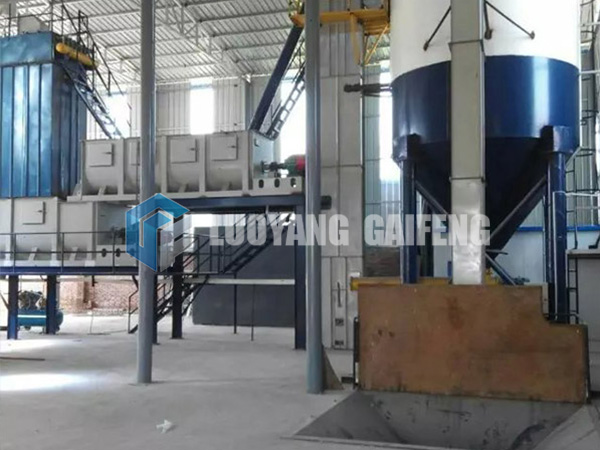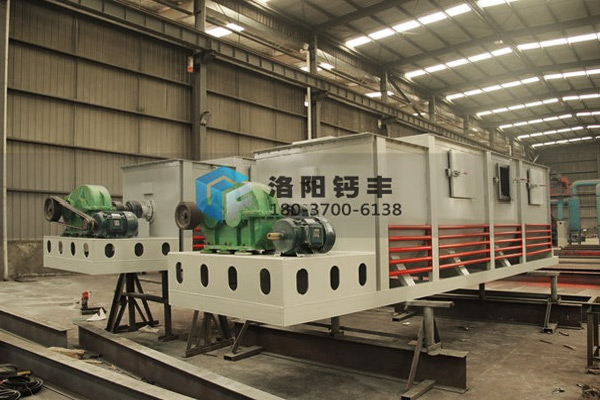# What is the relationship between the solubility of calcium hydroxide and temperature?

Calcium hydroxide is a substance that can be dissolved in water, but its solubility decreases as the temperature increases. Due to the particularity of the decrease in the solubility of calcium hydroxide with temperature changes, many people can’t help asking questions, causing calcium hydroxide The relationship between solubility and temperature What are the influencing factors of this state? With this question in mind, the calcium hydroxide production line manufacturer will introduce the relationship between the solubility of calcium hydroxide and temperature.### The relationship between calcium hydroxide solubility and temperature:

1. After a series of tests, we found that during the reaction process, a lot of heat was released, which made the temperature of the solution rise. During the whole reaction process, the mass of water in the solution decreases due to the consumption of part of the water.

2. Due to the decrease of the solvent in the solution and the increase of the solution temperature, part of the solute Ca(OH)2 is precipitated, which makes the quality of the solute in the solution decrease. After a while, as the temperature of the solution decreases, when the temperature of the solution returns to the original temperature, it still cannot reach the original quality.

3. The subtle changes in the quality of the solvent and the solute in the solution cause similar fluctuations in the quality of the solution. The quality of the solution first decreases and then increases a little bit. Compared with the beginning, the quality of the obtained solution is less than that before adding quicklime. the quality of the solution.

4. The temperature of the solution increases first and then decreases, and slowly returns to the original temperature. The obtained solution is still a saturated solution at the original temperature, so during the whole process, the solute mass fraction first decreases and then increases to the original value.5. This is because there are two processes when calcium hydroxide dissolves in water. First, the chemical bonds or intermolecular forces inside the substance are pulled apart. In this step, heat is absorbed, and then the pulled apart substance ions or molecules are separated from water or The water ions recombine, and heat is released in this step, most of which are soluble in water.

7. The energy released when calcium hydroxide combines with water is greater than the energy absorbed by dismantling the original structure of the substance in the previous step, so the result is that the total chemical energy of the system becomes lower, so the melting process can occur spontaneously.

8. The exothermic temperature of calcium hydroxide increased during the experiment, but there are a small number of substances like calcium hydroxide, the energy released by their combination with water is less than the energy absorbed by dismantling the original structure, but their melting process It can happen spontaneously, even when the temperature drops.

The above is the editor’s analysis of the relationship between the solubility and temperature of calcium hydroxide. The liquid state of calcium hydroxide is much more messy than the state of solid calcium hydroxide plus pure water. In other words, the solubility of calcium hydroxide solution is much higher, so although calcium hydroxide dissolves in water, the total chemical energy is increased, but it slows down during the dissolution process. High-purity calcium hydroxide is inseparable from the production of the entire production line